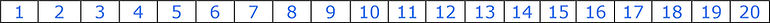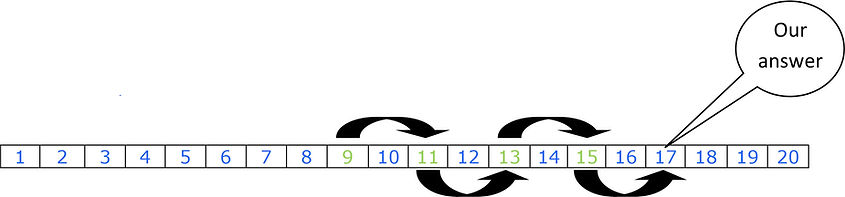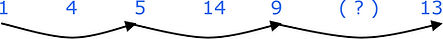# Question Type 11 Method Sheet

Type 11 – Number series

In this question type you are asked to insert the next number or numbers into the series.

Writing out the numbers in a way something like this can be very helpful as it will help you see. (Of course this is not practical if the numbers are very large.)Look at this first example and then we can work our way through the question until we get the answer: -

Find the next number in this series.

9        11      13      15      ( ? )

We can use our printed numbers to help us.

For ease of learning we have coloured the numbers used in the question in GREEN.
We have now drawn arrows from one number to the next number in the series because this will help us to see any pattern that there is in the series. As this is a very simple series we can see that the next number and our answer will 17 as we skip one number forward each time.

Look at this second example and then we can work our way through the question until we get the answer: -

Find the next number in this series.

1        4        9        16      ( ? )

We can use our printed numbers to help us.For ease of learning we have coloured the numbers used in the question in GREEN.
We have now drawn arrows from one number to the next number in the series because this will help us to see any pattern that there is in the series.
This is a more complicated series and we can see that our last arrow points towards question marks. This series requires you to realise that this series is dealing with
“square numbers” so the next number and our answer will be 25 as we have 1 squared, then 2 squared, 3 squared, 4 squared and finally 5 squared to give us 25 which is our answer.
(When a number is squared it is multiplied by itself.
It can also be written like this
which means 3 multiplied by 3.)

Look at this third example and then we can work our way through the question until we get the answer: -

Find the next number in this series.

1        4        5        14      9        ( ? )

We can use our printed numbers to help us.For ease of learning we have coloured the numbers used in the question in GREEN.
We have now drawn arrows from one number to the next number in the series because this will help us to see any pattern that there is in the series.

We can see that the series goes from

1 to 4(plus 3) then to 5(plus 1) then to 14(plus 9)  and finally to 9 (minus 5 )!!

There does not appear to be any really clear series in this question!
This is a more complicated series and what we have are actually two series combined.Series one:- 1 to 5(plus 4) then 5 to 9(plus 4) and the next number would be 13
ie 9 to 13(plus 4) !!but this is not our answer!!

Series two:- 4 to 14(plus 10) then 14 to our answer by adding 10 which is 24!!
(When you are given 5 or more numbers in the question there may be two or more series combined in the question.)

THINGS TO LOOK OUT FOR!!!!

Writing out the numbers may be very useful.
Drawing on the series/numbers will help you to spot the series.
Draw above and below the series/numbers if necessary.
Check to see if the series is going forwards, backwards or is a mixture of two or more series.
Check the series and ensure that you have counted properly between the numbers.
This question type is similar to series questions using letters rather than numbers.
Make sure that you work systemically.
Avoid guessing the answer.

There are many different series and below we give some examples.

NB When you are completing a Verbal Reasoning test in Multiple-Choice format the correct answer will always be one of the choices that you are given on your answer sheet. If your answer does not match one of the answers that you are given then your answer is wrong.